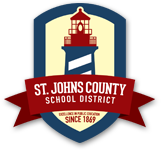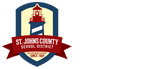• News
• Careers
• Calendar
• Home Access Center (HAC)
• Contact

# Mathematics

### Algebra 1 Honors

Course Number:
1200320
Credit:
1.00
Weight:
Honors 0.5
Term:
Yearlong
Prerequisite:
None
Course Description:

This course is a rigorous study designed for the student who excels in both ability and performance in mathematics. The critical areas of this course deepen and extend understanding of the number system and of linear and exponential relationships by contrasting them with each other and by applying linear models to statistical data that exhibit a linear trend, and students engage in methods for analyzing, solving, and using quadratic functions. The standards for these critical areas fall into three reporting categories: Algebra and Modeling, Functions and Modeling, and, Statistics and the Number System. The Standards for Mathematical Practice apply throughout each course and, together with the content standards, prescribe that students experience mathematics as a coherent, useful, and logical subject that makes use of their ability to make sense of real-world scenarios. Students must participate in the End-of-Course examination.

### Geometry Honors

Course Number:
1206320
Credit:
1.00
Weight:
Honors 0.5
Term:
Yearlong
Prerequisite:
None
Course Description:

This course is designed for the student who excels in both ability and performance in college preparatory mathematics. This is a rigorous study in which students will explore more complex geometric situations and deepen their explanations of geometric relationships, moving towards formal mathematical arguments. The standards for this course fall into three critical areas (reporting categories): Congruence, Similarity, Right Triangles and Trigonometry, Circles, Geometric Measurement and Geometric Properties with Equations, and, Modeling with Geometry. The Standards for Mathematical Practice apply throughout each course and, together with the content standards, prescribe that students experience mathematics as a coherent, useful, and logical subject that makes use of their ability to make sense of real-world scenarios. Extensive out-of-class preparation is required. This course emphasizes the relationship between Algebra and Geometry in preparation for Algebra 2 Honors.

### M/J Accelerated Mathematics Grade 6

Course Number:
1205020
Credit:
0.00
Weight:
Standard 0.0
Term:
Yearlong
Prerequisite:
None
Course Description:

The purpose of this course is to focus on four critical areas: (1) connecting ratio and rate to whole number multiplication and division and using concepts of ratio and rate to solve problems, (2) completing understanding of division of fractions and extending the notion of number to the system of rational numbers, which includes negative numbers, (3) writing, interpreting, and using expressions and equations, (4) developing understanding of statistical thinking, (5) developing understanding of and applying proportional relationships, and (6) developing understanding of operations with rational numbers and working with expressions and linear equations.

### M/J Accelerated Mathematics Grade 7

Course Number:
1205050
Credit:
0.00
Weight:
Standard 0.0
Term:
Yearlong
Prerequisite:
None
Course Description:

The purpose of this course is to focus on five critical areas: (1) solving problems involving scale drawings and informal geometric constructions, and working with two- and three-dimensional shapes to solve problems involving area, surface area, and volume, (2) drawing inferences about populations based on samples, (3) formulating and reasoning about expressions and equations, including modeling an association in bivariate data with a linear equation, and solving linear equations and systems of linear equations, (4) grasping the concept of a function and using functions to describe quantitative relationships, and (5) analyzing two- and three-dimensional space and figures using distance, angle, similarity, and congruence, and understanding and applying the Pythagorean Theorem.

Course Number:
1205010
Credit:
0.00
Weight:
Standard 0.0
Term:
Yearlong
Prerequisite:
None
Course Description:

The purpose of this course is to focus on four critical areas: (1) connecting ratio and rate to whole number multiplication and division and using concepts of ratio and rate to solve problems, (2) completing understanding of division of fractions and extending the notion of number to the system of rational numbers, which includes negative numbers, (3) writing, interpreting, and using expressions and equations, (4) developing understanding of statistical thinking.

Course Number:
1205040
Credit:
0.00
Weight:
Standard 0.0
Term:
Yearlong
Prerequisite:
None
Course Description:

The purpose of this course is to focus on four critical areas: (1) developing understanding of and applying proportional relationships, (2) developing understanding of operations with rational numbers and working with expressions and linear equations, (3) solving problems involving scale drawings and informal geometric constructions, and working with two- and three-dimensional shapes to solve problems involving area, surface area, and volume, and (4) drawing inferences about populations based on samples.

Course Number:
1205070
Credit:
0.00
Weight:
Standard 0.0
Term:
Yearlong
Prerequisite:
None
Course Description:

The purpose of this course is to focus on three critical areas: (1) formulating and reasoning about expressions and equations, including modeling an association in bivariate data with a linear equation, and solving linear equations and systems of linear equations, (2) grasping the concept of a function and using functions to describe quantitative relationships, (3) analyzing two- and three-dimensional space and figures using distance, angle, similarity, and congruence, and understanding and applying the Pythagorean Theorem.

### M/J Intensive Mathematics (MC)

Course Number:
1204000
Credit:
0.00
Weight:
Standard 0.0
Term:
Yearlong
Prerequisite:
None
Course Description:

The purpose of this course is to enable students to develop mathematics skills and concepts through remedial instruction and practice. The content should include mathematics content that has been identified by screening and individual diagnosis of each student’s need for remedial instruction as specified in his/her progress monitoring intervention plan.

### M/J MATH 1 CLS

Course Number:
1205030
Credit:
0.00
Weight:
Standard 0.0
Term:
Yearlong
Prerequisite:
None
Course Description:

Math

### M/J Math 2 Cambridge Lower Secondary

Course Number:
1205055
Credit:
0.00
Weight:
Standard 0.0
Term:
Yearlong
Prerequisite:
None
Course Description:

Math

### M/J Mathematics 3 Cambridge

Course Number:
1205060
Credit:
0.00
Weight:
Standard 0.0
Term:
Yearlong
Prerequisite:
None
Course Description:

Math

© 2023 St. Johns County School District